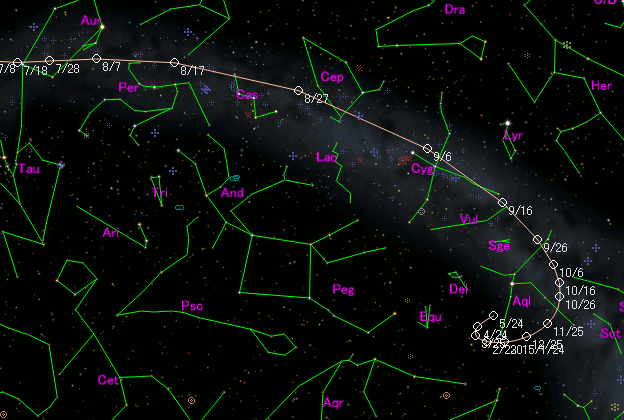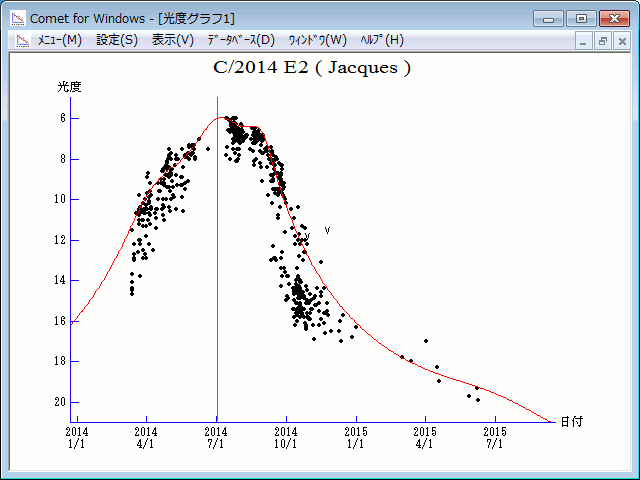# \$B%8%c%C%/WB@1(B

C/2014 E2 ( Jacques )###\$B%W%m%U%#!<%k(B

 \$BH/8+F|(B 2014\$BG/(B3\$B7n(B13\$BF|(B \$BH/8+8wEY(B 14.7\$BEy(B \$BH/8+ Cristovao Jacques (SONEAR Observatory near Oliveira, Brazil)

###\$B###\$B50F;MWAG(B

```Epoch 2014 July 2.0 TT = JDT 2456840.5
T 2014 July 2.51570 TT                                  MPCW
q   0.6639310            (2000.0)            P               Q
z  +0.0014614      Peri.  344.04135     +0.32236742     +0.88590997
Node    56.39125     +0.90640511     -0.39047951
e   0.9990298      Incl.  156.39209     +0.27296341     +0.25037828
From 4583 observations 2014 Mar. 13-2015 June 8, mean residual 0".5.
Nongravitational parameters A1 = +2.09, A2 = -0.2798.
```

###\$B@1?^(B###\$B8wEYJQ2=(B

```        m1 = 6.0 + 5 log\$B&\$(B + 15.0 log r  [   ,-63]  (              \$B!A(B2014\$BG/(B 4\$B7n(B30\$BF|(B)
m1 = 6.7 + 5 log\$B&\$(B + 10.0 log r  [-63, 63]  (2014\$BG/(B 4\$B7n(B30\$BF|!A(B2014\$BG/(B 9\$B7n(B 3\$BF|(B)
m1 = 6.0 + 5 log\$B&\$(B + 15.0 log r  [ 63,   ]  (2014\$BG/(B 9\$B7n(B 3\$BF|!A(B              )
```##### \$B50F;MWAG\$O(BM.P.E.C. 2016-D32\$B\$K7G:\\$5\$l\$?\$b\$N\$G\$9!#(B \$B@1?^\$O%9%F%i%J%S%2!<%?(B Ver.8 (\$B%"%9%H%m%"!<%D(B) \$B\$G:n@.\$7\$?\$b\$N\$G\$9!#(B \$B8wEY%0%i%U\$O(BComet for Windows\$B\$G:n@.\$7\$?\$b\$N\$G\$9!#(B